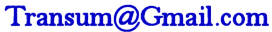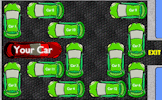# Fractions

## A series of self-marking exercises on adding, subtracting, multiplying and dividing fractions.

##### Level 1Level 2Level 3Level 4Level 5Level 6Level 7Level 8Level 9Mixed NumbersDescriptionHelpMore

This is level 1: adding two fractions with the same denominators. Give your answers as single fractions in their lowest terms. You can earn a trophy if you get at least 9 correct and you do this activity online.

$$\frac{1}{3} + \frac{1}{3}$$
 =$$\frac{2}{4} + \frac{1}{4}$$
 =$$\frac{2}{5} + \frac{1}{5}$$
 =$$\frac{1}{6} + \frac{3}{6}$$
 =$$\frac{2}{7} + \frac{1}{7}$$
 =$$\frac{3}{8} + \frac{4}{8}$$
 =$$\frac{1}{9} + \frac{2}{9}$$
 =$$\frac{4}{10} + \frac{5}{10}$$
 =$$\frac{5}{11} + \frac{5}{11}$$
 =$$\frac{2}{12} + \frac{3}{12}$$
 =$$\frac{6}{13} + \frac{3}{13}$$
 =$$\frac{7}{14} + \frac{4}{14}$$
 =Check

This is Fractions level 1. You can also try:
Level 2 Level 3 Level 4 Level 5 Level 6 Level 7 Level 8 Level 9

You can also try the Mixed Numbers exercises: Level 1 Level 2 Level 3 Level 4

## Instructions

Try your best to answer the questions above. Type your answers into the boxes provided leaving no spaces. As you work through the exercise regularly click the "check" button. If you have any wrong answers, do your best to do corrections but if there is anything you don't understand, please ask your teacher for help.

When you have got all of the questions correct you may want to print out this page and paste it into your exercise book. If you keep your work in an ePortfolio you could take a screen shot of your answers and paste that into your Maths file.

## Transum.org

This web site contains over a thousand free mathematical activities for teachers and pupils. Click here to go to the main page which links to all of the resources available.## More Activities:

Mathematicians are not the people who find Maths easy; they are the people who enjoy how mystifying, puzzling and hard it is. Are you a mathematician?

Comment recorded on the 17 June 'Starter of the Day' page by Mr Hall, Light Hall School, Solihull:

"Dear Transum,

I love you website I use it every maths lesson I have with every year group! I don't know were I would turn to with out you!"

Comment recorded on the 1 May 'Starter of the Day' page by Phil Anthony, Head of Maths, Stourport High School:

"What a brilliant website. We have just started to use the 'starter-of-the-day' in our yr9 lessons to try them out before we change from a high school to a secondary school in September. This is one of the best resources on-line we have found. The kids and staff love it. Well done an thank you very much for making my maths lessons more interesting and fun."

#### Car Park PuzzleCan you get your car out of the very crowded car park by moving other cars forwards or backwards? There are five levels of increasing difficulty and the interactive interface makes this a fun problem solving exercise.

There are answers to this exercise but they are available in this space to teachers, tutors and parents who have logged in to their Transum subscription on this computer.

A Transum subscription unlocks the answers to the online exercises, quizzes and puzzles. It also provides the teacher with access to quality external links on each of the Transum Topic pages and the facility to add to the collection themselves.

Subscribers can manage class lists, lesson plans and assessment data in the Class Admin application and have access to reports of the Transum Trophies earned by class members.

Subscribe

## Go Maths

Learning and understanding Mathematics, at every level, requires learner engagement. Mathematics is not a spectator sport. Sometimes traditional teaching fails to actively involve students. One way to address the problem is through the use of interactive activities and this web site provides many of those. The Go Maths page is an alphabetical list of free activities designed for students in Secondary/High school.

## Maths Map

Are you looking for something specific? An exercise to supplement the topic you are studying at school at the moment perhaps. Navigate using our Maths Map to find exercises, puzzles and Maths lesson starters grouped by topic.

## Teachers

If you found this activity useful don't forget to record it in your scheme of work or learning management system. The short URL, ready to be copied and pasted, is as follows:

Do you have any comments? It is always useful to receive feedback and helps make this free resource even more useful for those learning Mathematics anywhere in the world. Click here to enter your comments.For All:

© Transum Mathematics :: This activity can be found online at:
www.transum.org/software/SW/Starter_of_the_day/Students/Fractions.asp?Level=1

## Description of Levels

CloseLevel 1 - Adding two fractions with the same denominators.

Level 2 - Adding two fractions with a common denominator.

Level 3 - Adding two fractions with random denominators.

Level 4 - Subtracting two fractions with the same denominators.

Level 5 - Subtracting two fractions with a common denominator.

Level 6 - Subtracting two fractions with random denominators.

Level 7 - Multiplying two fractions.

Level 8 - Dividing one fraction by another.

Level 9 - Mixed fraction calculations.

Mixed Numbers exercises.

Answers to this exercise are available lower down this page when you are logged in to your Transum account. If you don’t yet have a Transum subscription one can be very quickly set up if you are a teacher, tutor or parent.

You may also want to use a calculator to check your working. See Calculator Workout skill 10.

Don't wait until you have finished the exercise before you click on the 'Check' button. Click it often as you work through the questions to see if you are answering them correctly.

Answers to this exercise are available lower down this page when you are logged in to your Transum account. If you don’t yet have a Transum subscription one can be very quickly set up if you are a teacher, tutor or parent.

Close